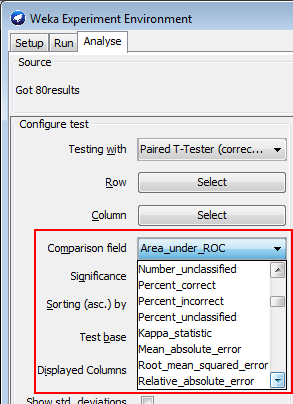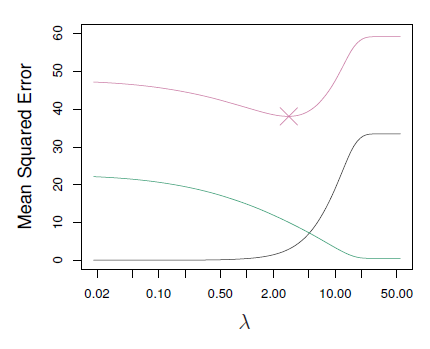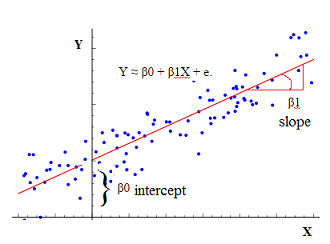# Statistics - R-squared ( |Coefficient of determination) for Model Accuracy

$R^2$ is an accuracy statistics in order to assess a regression model. It's a summary of the model.

$R^2$ is the percentage of variance in Y explained by the model, the higher, the better.

The largest r squared is equivalent to the smallest residual sum of squares.

R squared is also known as:

• the fraction of variance explained.
• the sum of squares explained.
• Coefficient of determination

It's a way to compare competing models.

R squared: two same definitions with two different formulations:

• R squares tells us the proportion of variance in the outcome measure that is explained by the predictors
• or The predictor explains (R squared) percentage of the variance in the outcome measure.

If R Squared increases the models get better.

Example by adding multiple predictor if R Squared increased, we say that the model is boosted.

r squared tells the proportion of variance explained by a linear regression model, by a least squares model.

## Formula

$$\begin{array}{rrl} R^2 & = & 1 - \frac{\href{RSS}{RSS}}{TSS} \\ TSS & = & \sum^N_{i=0} (y_i - \bar{y})^2 \\ \end{array}$$

• TSS = Total Sum of Squares where $y_i$ is the i-th response and $\bar{y}$ is the average response.

## Documentation / Reference

Recommended PagesData Mining - (Parameters | Model) (Accuracy | Precision | Fit | Performance) Metrics

Accuracy is a evaluation metrics on how a model perform. rare event detection Hypothesis testing: t-statistic and p-value. The p value and t statistic measure how strong is the...R - Feature Selection - Indirect Model Selection

In a feature selection process, once you have generated all possible models, you have to select the best one. This article talks the indirect methods. We will select the models using CP but as...R - Feature selection - Model Generation (Best Subset and Stepwise)

This article talks the first step of feature selection in R that is the models generation. Once the models are generated, you can select the best model with one of this approach: Best subset regressiosigreedy...R - Interaction Analysis

interaction with R . An interaction term between a numeric x and z is just the product of x and z. lm processes the “” operator between variables andautomatically: add the interaction...R - Multiple Linear Regression

Multiple linear regression with R functions such as lm Unstandardized Multiple Regression Regression analyses, standardized (in the z scale). The point is a short-cut to select all variables....R - Shrinkage Method (Ridge Regression and Lasso)

Unlike subset and forward stepwise regression, which controls the complexity of a model by restricting the number of variables, ridge regression keeps all the variables in and shrinks the coefficients...Statistics - (Interaction|Synergy) effect

In a multiple regression, is assumed that the effect on the target of increasing one unit of one predictor (is independent|has no influence) on the other predictor. If this is not the case, sharing a...Statistics - (Shrinkage|Regularization) of Regression Coefficients

Shrinkage methods are more modern techniques in which we don't actually select variables explicitly but rather we fit a model containingall p predictors using a technique that constrains or regularizes...Statistics - (Univariate|Simple|Basic) Linear Regression

A Simple Linear regression is a linear regression with only one predictor variable (X). Correlation demonstrates the relationship between two variables whereas a simple regression provides an equation...